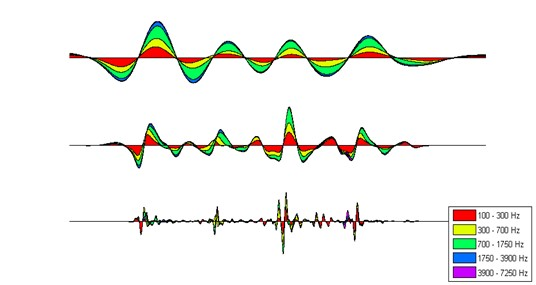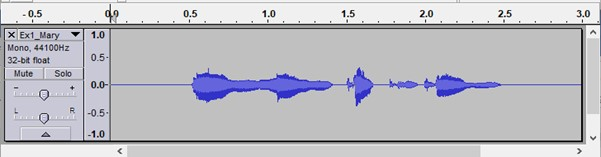Centre for Neuroscience in Education

The following MATLAB code should plot the 3 x 5 SAMPH matrix of the example soundfile “Mary Mary quite contrary”,  in the format as shown below.

figure

for m = 1:3 subplot(3,1,m);

area(data), colormap hsv

axis tight, axis off

end

## figure5_plot_s-amph_matrix_ta.jpgWaveform of “Mary Mary quite Contrary”

## figure4_plot_s-amph_matrix_ta.jpg